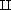Digital & Computing

### Become an OU studentData and processes in computing

Start this free course now. Just create an account and sign in. Enrol and complete the course for a free statement of participation or digital badge if available.

# Conclusion

1. We discussed forms of data and processes relevant to an electronic till in a supermarket. In particular, we introduced the idea of a sequence of data items.

2. A number of fundamental forms of data were introduced. We distinguished two types of number: integers (positive or negative whole numbers, or 0), and real numbers (thought of as decimal numbers and approximated in computers as floating point numbers). Characters may be thought of as symbols that may be entered from a computer keyboard by a single keystroke. Each character is associated with an integer code and we introduced one such encoding called the ASCII code. The Boolean values true and false form another fundamental form of data.

Data may be structured in a collection. Different forms of collection are possible. We looked at two: sets and sequences. In a sequence, the order in which the items appear in the collection is important and an item may appear more than once. In a set, one is only interested in the different items appearing in the collection, and the order in which these items are listed is of no significance, nor is repetition of an item.

The Cartesian product of sets X and Y is written X × Y , and is the set consisting of all ordered pairs (x, y), where x is in X and y is in Y . An ordered pair is also called a 2-tuple. More generally, an n-tuple is an association of n items, each taken from a specified set. An n-tuple is written in round brackets, and is a member of a Cartesian product set combining n component sets. For example, a member of the set Int × Char × Int × SeqOfChar would be a 4-tuple, such as (121, ‘y’, 6, “Qwerty”).

3. The disjoint union of sets X and Y, written XY, consists of all items that come either from X or from Y (where X and Y have nothing in common). This is useful when we want to mix different forms of data in a sequence.

4. A function is a process that returns a unique value for each permitted input. To give a full description of a function, we give: its signature (name, and input and output sets), a precondition (which may be true), and a postcondition giving its semantics. The semantics should be an unambiguous statement of the relationship between the returned value and the input(s).

If a function has more than one input, then its input set will be a Cartesian product. If a function returns a value that is true or false, then the output set will be Bool. Usually, the semantics of a function are given by a general rule, or possibly by a formula. However, in some cases we need to list the returned value for each possible input. For example, this would be the case for a function returning the price of each barcoded product stocked by a supermarket.

5. A binary operation is a process, such as addition of integers, that inputs two data items of the same type. A binary operation can be written using infix notation (as in x + y, for example). Addition of integers corresponds to a function with signature Int × IntInt. We described integer division. Integer division of n by d returns an integer, written DIV (n, d). It also leaves a remainder, denoted by MOD(n, d).

We noted that binary operations are not confined to those on numbers. We described two binary operations on Boolean values that are frequently useful: ∨ (read as “or”) and ∧ (read as “and”). We also introduced the function NOT on Booleans.

Comparisons, such as < or =, are operations returning values in the output set Bool. For example, =Char , a test of whether two characters are identical, corresponds to a function with signature Char × CharBool.